# What Is The Second Law Of Thermodynamics?

The second law of thermodynamics states that, in a spontaneous process, the total entropy of the universe always increases.

Can a heat engine operate at 100% efficiency? In the year 1824, scientist Nicolas Léonard Sadi Carnot, also known as the father of thermodynamics, tried to find the answer. In one of his theoretical models, he placed a cylinder with a piston between two heat reservoirs. Both the reservoirs were at a constant temperature, but one had a temperature higher than the other.

The idea was to convert the heat flow between the two reservoirs into work. To do that, he placed a cylinder with a piston between the reservoirs. However, he realized that it was impossible to achieve 100% efficiency. Some heat always flowed through the cylinder to the cold reservoir.

The first law of thermodynamics states that energy can neither be created nor destroyed; it can only change forms. However, it has certain limitations. It does not define the direction in which a change can happen, nor does it tell if a change is reversible or not. If we drop an object from a height, the potential energy is converted to kinetic energy, and it falls. However, after hitting the ground, it does not bounce back to the same height. Why is that the case? To understand this, let’s look at a critical term: Spontaneous Process.

Recommended Video for you:

## Spontaneous Process

A spontaneous process in thermodynamics is one that can happen without any external intervention. It must also have enough time to happen. Put a drop of ink in a glass of water and it spreads throughout the glass “spontaneously”, whereas a spoonful of sugar needs some stirring to dissolve into a glass of water. When it’s hot outside, your room is heated “spontaneously”, but it takes an air conditioner to keep your room cold.Ink spreads in water spontaneously

By experience, we know that some events that happen in our lives are spontaneous. However, scientists needed a way to define the spontaneity of any event. They needed a way to define the direction in which a change could happen. This need gave birth to what we know as the second law of thermodynamics.

## The Second Law of Thermodynamics

The laws of thermodynamics define how work, heat, and energy affect a given system. A system is any region in the universe that is finitely bounded, across which energy is transferred. Everything outside this boundary is considered its surroundings.(Photo Credit : Wavesmikey / Wikipedia Commons)

The second law of thermodynamics states that, in a spontaneous process, the total entropy of the universe always increases. It is also defined using the formula: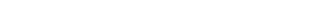Here, S denotes the entropy of the system. To understand this formula, let’s look at the term: Entropy.

## Entropy

If you’ve ever heard of this term, you may associate it with “disorder.” However, in thermodynamics, the definition is more evolved.

Before you can properly understand Entropy, you must understand that energies are quantized. When electrons in an atom get energy, they absorb it only in integral multiples of a small quantity of energy called a “quantum”.

When you put a drop of ink in a glass of water, it spreads throughout the water. Similarly, if you have a hot metal kept on a table, the heat from it spreads across the environment. Imagine that there is a box containing 5 molecules of gas. It is kept right next to this hot metal. The metal transfers 5 quanta of heat to the gas. Will each molecule of gas get 1 quantum of energy each? Not necessarily.It is possible that 2 molecules will get 2 quanta each and one molecule will get 1 quantum, while the other 2 units will not get any. It is also possible that one molecule will get 3 quanta, another will get 2, and the others will get none. There can be 126 possible combinations, which can be calculated using the formula: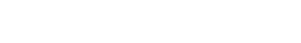Here, N is the number of molecules and q is the quanta of energy provided.

These molecules can also bump into each other and exchange their kinetic energy. However, the total energy cannot exceed the amount of energy provided.

Each of these combinations is called a “microstate”. The total energy level is called a “macrostate”. The entropy is a measure of the number of ways that energy can be distributed to all the molecules. Thus, it is a factor of the number of “microstates”, also represented as Ω.Entropy is a state variable. A state variable is one that describes the physical state of a system, like pressure, temperature and volume. The mathematical formula of entropy is:Here, kB is the Boltzmann constant. It is a physical constant that signifies the average kinetic energy of particles in a gas relating to the temperature. This constant is multiplied to the natural log of the number of microstates to calculate entropy.

The change in entropy can also be calculated by dividing the heat absorbed by the temperature. The heat absorbed increases the kinetic energy of the particles. Thus, the formula is:In a nutshell, entropy is a measure of the number of ways that energy can be distributed to a set of molecules in a system.

## Calculating the Entropy of a Heat Engine

Let’s go back to Carnot’s engine and try to figure out when a heat engine is possible. Let’s assume that the hot reservoir has a temperate of TH and the cold reservoir has a temperature of TC. A QH amount of heat is extracted from the hot reservoir to do some work.Thus, the change in entropy of the hot reservoir is:Q is negative because heat is extracted from the reservoir

As the piston of the engine does the work and comes back to its initial state, the change in entropy of the engine () is 0.

If we assume that all the energy is used up to do the work and no heat is given to the cold reservoir, then the entropy of the cold reservoir () is also 0.

So,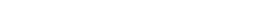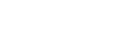The total entropy of the universe becomes negative, and thus it cannot be spontaneous.

Now, let’s assume the cold reservoir absorbs a QC amount of heat and is not used to do any work. In that case, the entropy of the cold reservoir () isIn that case,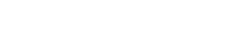The only case the engine can work is when theis positive oris positive, as stated by the second law of thermodynamics.

In the late 19th century, Lord Kelvin and Max Plank had these observations. They stated: “One cannot convert a quantity of heat completely into work without wasting some of this heat at a lower temperature.” This statement is also an alternate statement for the second law of thermodynamics.

## Universal Law

Albert Einstein was quite fluent in the complex language of thermodynamics. In fact, he published several papers on the subject. He once quoted: “A theory is more impressive the greater the simplicity of its premises, the more different kinds of things it relates, and the more extended its area of applicability. Therefore, the deep impression that classical thermodynamics made upon me. It is the only physical theory of universal content which I am convinced will never be overthrown, within the framework of applicability of its basic concepts.”

The second law of thermodynamics drives the universe. We know that the universe is expanding. As per the second law of thermodynamics, the entropy of the universe must also increase. Stars are the source of energy for the universe. By the time the last star emits its final photon, the level of entropy will be incredibly high. Don’t worry… we all would have perished long before this time. That is when the universe will die, as there will be no energy left to increase the entropy of the universe!

What is The First Law of Thermodynamics?

What is The Third Law of Thermodynamics?